# clusterDBSCAN.estimateEpsilon

Estimate neighborhood clustering threshold

## Syntax

``epsilon = clusterDBSCAN.estimateEpsilon(X,MinNumPoints,MaxNumPoints)``
``clusterDBSCAN.estimateEpsilon(X,MinNumPoints,MaxNumPoints)``

## Description

example

````epsilon = clusterDBSCAN.estimateEpsilon(X,MinNumPoints,MaxNumPoints)` returns an estimate of the neighborhood clustering threshold, `epsilon`, used in the density-based spatial clustering of applications with noise (DBSCAN)algorithm. `epsilon` is computed from input data `X` using a k-nearest neighbor (k-NN) search. `MinNumPoints` and `MaxNumPoints` set a range of k-values for which epsilon is calculated. The range extends from `MinNumPoints` – 1 through `MaxNumPoints` – 1. k is the number of neighbors of a point, which is one less than the number of points in a neighborhood.```
````clusterDBSCAN.estimateEpsilon(X,MinNumPoints,MaxNumPoints)` displays a figure showing the k-NN search curves and the estimated epsilon.```

## Examples

collapse all

Create simulated target data and use the `clusterDBSCAN.estimateEpsilon` function to calculate an appropriate epsilon threshold.

Create the target data as xy Cartesian coordinates.

```X = [randn(20,2) + [11.5,11.5]; randn(20,2) + [25,15]; ... randn(20,2) + [8,20]; 10*rand(10,2) + [20,20]];```

Set the range of values for the k-NN search.

```minNumPoints = 15; maxNumPoints = 20;```

Estimate the clustering threshold epsilon and display its value on a plot.

`clusterDBSCAN.estimateEpsilon(X,minNumPoints,maxNumPoints)`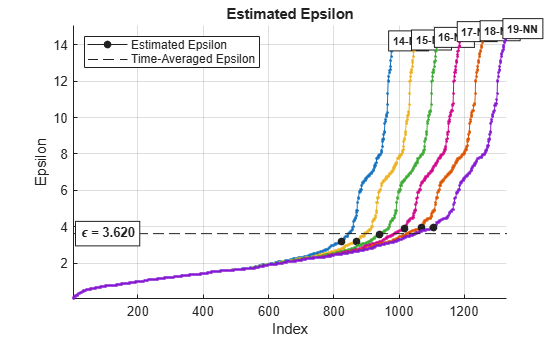Use the estimated Epsilon value, 3.62, in the `clusterDBSCAN` clusterer. Then, plot the clusters.

```clusterer = clusterDBSCAN('MinNumPoints',6,'Epsilon',3.62, ... 'EnableDisambiguation',false); [idx,cidx] = clusterer(X); plot(clusterer,X,idx)```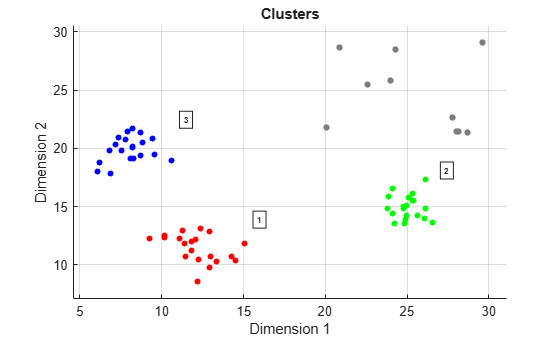## Input Arguments

collapse all

Input feature data, specified as a real-valued N-by-P matrix. The N rows correspond to feature points in a P-dimensional feature space. The P columns contain the values of the features over which clustering takes place. The DBSCAN algorithm can cluster any type of data with appropriate `MinNumPoints` and `Epsilon` settings. For example, a two-column input can contain the xy Cartesian coordinates, or range and Doppler.

Data Types: `double`

The starting value of the k-NN search range, specified as a positive integer. `MinNumPoints` is used to specify the starting value of k in the k-NN search range. The starting value of k is one less than `MinNumPoints`.

Example: `10`

Data Types: `double`

The end value of k-NN search range, specified as a positive integer. `MaxNumPoints` is used to specify the ending value of k in the k-NN search range. The ending value of k is one less than `MaxNumPoints`.

## Output Arguments

collapse all

Estimated epsilon, returned as a positive scalar.

## Algorithms

collapse all

### Estimate Epsilon

DBSCAN clustering requires a value for the neighborhood size parameter ε. The `clusterDBSCAN` object and the `clusterDBSCAN.estimateEpsilon` function use a k-nearest-neighbor search to estimate a scalar epsilon. Let D be the distance of any point P to its kth nearest neighbor. Define a Dk(P)-neighborhood as a neighborhood surrounding P that contains its k-nearest neighbors. There are k + 1 points in the Dk(P)-neighborhood including the point P itself. An outline of the estimation algorithm is:

• For each point, find all the points in its Dk(P)-neighborhood

• Accumulate the distances in all Dk(P)-neighborhoods for all points into a single vector.

• Sort the vector by increasing distance.

• Plot the sorted k-dist graph, which is the sorted distance against point number.

• Find the knee of the curve. The value of the distance at that point is an estimate of epsilon.

The figure here shows distance plotted against point index for k = 20. The knee occurs at approximately 1.5. Any points below this threshold belong to a cluster. Any points above this value are noise.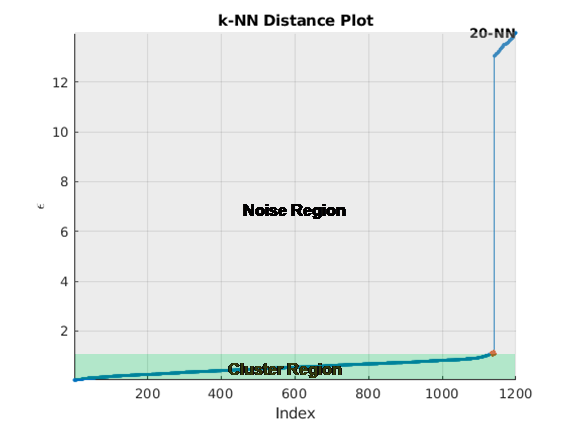There are several methods to find the knee of the curve. `clusterDBSCAN` and `clusterDBSCAN.estimateEpsilon` first define the line connecting the first and last points of the curve. The ordinate of the point on the sorted k-dist graph furthest from the line and perpendicular to the line defines epsilon.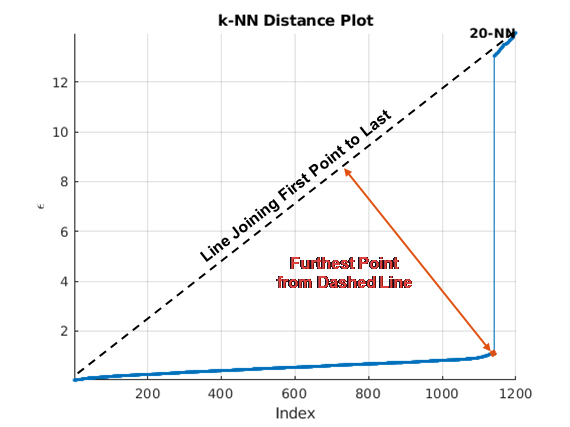When you specify a range of k values, the algorithm averages the estimate epsilon values for all curves. This figure shows that epsilon is fairly insensitive to k for k ranging from 14 through 19.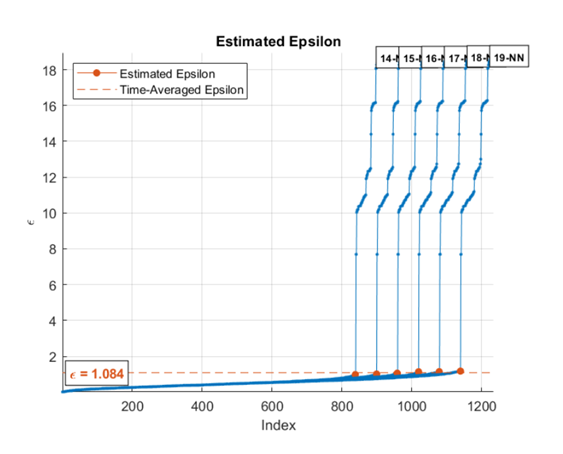To create a single k-NN distance graph, set the `MinNumPoints` property equal to the `MaxNumPoints` property.

### Choosing the Minimum and Maximum Number of Points

The purpose of `MinNumPoints` is to smooth the density estimates. Because a cluster is a maximal set of density-connected points, choose smaller values when the expected number of detections in a cluster is unknown. However, smaller values make the DBSCAN algorithm more susceptible to noise. A general guideline for choosing `MinNumPoints` is:

• Generally, set `MinNumPoints` = 2P where P is the number of feature dimensions in `X`.

• For data sets that have one or more of the following properties:

• many noise points

• large number of points, `N`

• large dimensionality, `P`

• many duplicates

increasing `MinNumPoints` can often improve clustering results.

## Extended Capabilities